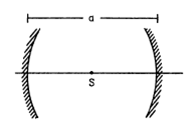Deepak Scored 45->99%ile with Bounce Back Crack Course. You can do it too!

# A point source S is placed midway between two converging

Question:

A point source $S$ is placed midway between two converging mirror having equal focal length $f$ as shown in fig. Find the values od d for which only one image is formed.Solution:

Both mirror produces one image in following two cases:

(A) When Source is at distance $=2 f$ or at center of curvature then image is produce at $v=2 f+2 f=4 f$

(B) When source is at distance $f$ then rays become parallel after reflecting through one mirror, then it is reflected through other mirror and only one image is formed

$V=f+f=2 f$